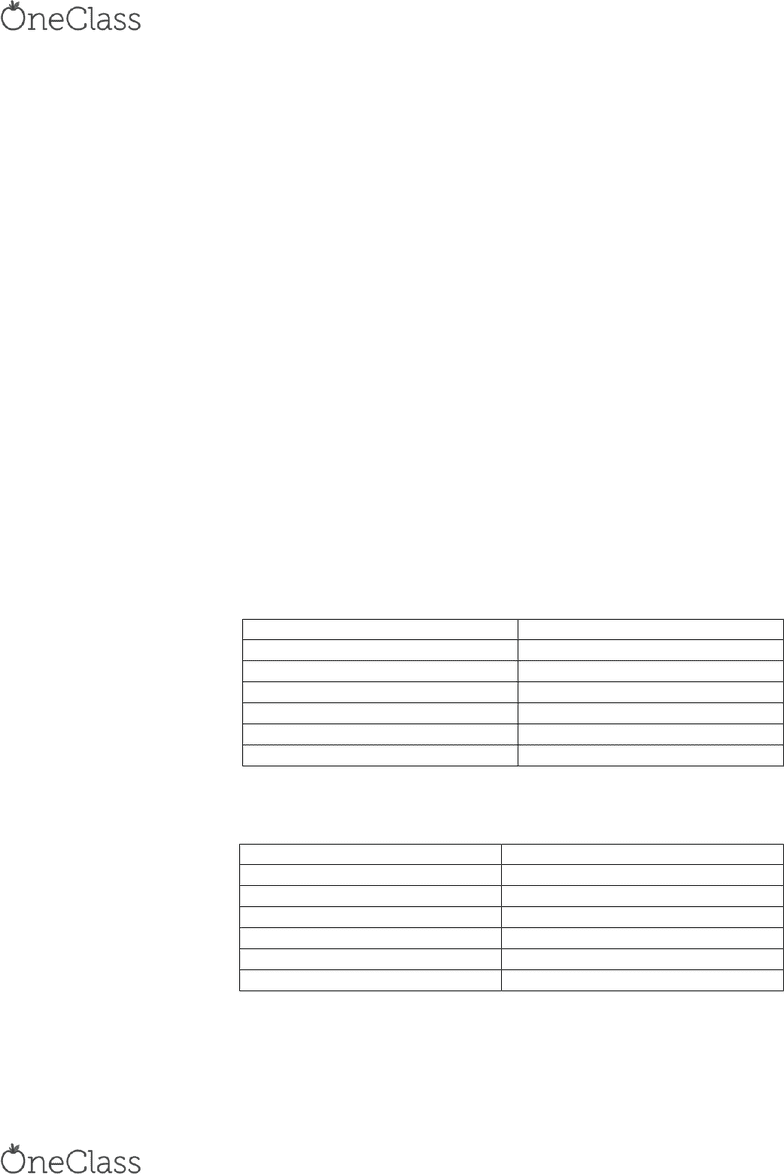# ENGG 311 Lecture Notes - Lecture 2: Thermodynamics, Kilogram, Carbon-12

38 views4 pagesFROM LECTURE 1:
a practice quiz is available online
The midterm will be held on March 3 and is 2 hours long
The tetook is Fudaetals of Egieerig Therodais, Mora et. al., 8th ed.
An older edition of the textbook is acceptable, but steam tables must be purchased from the
bookstore.
Steam tables are necessary for the exams. The proper version is in the back of the current
edition of the textbook, and they are available for \$18 from the bookstore
Lab groups are in the Lab schedule document on D2L
ENGG Lecture
Thermodynamics is the study of heat and power
Terms:
Dimension: A measurable property that cannot be defined in terms of anything simpler
o Primary: The most basic dimension that cannot be reduced to anything simpler
Length [L], Mass [M], Time [t], Temperature [θ], Amount of material [n]
o Derived: Dimensions that can be defined in terms of primary dimensions
Velocity (Lt-1), acceleration (Lt-2), Force (MLt-2), Work (ML2t-2), Power (ML2t-3)
Unit: A way of expressing a dimension.
o In SI:
Length
Meter (m)
Mass
Kilogram (kg)
Time
Second (s)
Temperature
Degree Celsius (°C)
Absolute Temperature
Kelvin (K)
Amount of Material
Mole
Force
Newton (N)
o In English Engineering:
Length
Foot (ft)
Mass
Pound Mass (lb)
Time
Second (s)
Temperature
Degree FahrenheitF)
Absolute Temperature
Degree Rankine (°R)
Amount of Material
Mole
Force
Pound Force (lbf)
find more resources at oneclass.com
find more resources at oneclass.com
Unlock document

This preview shows page 1 of the document.
Unlock all 4 pages and 3 million more documents.